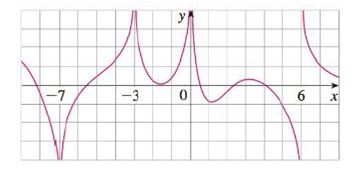Chapter 2.2, Problem 9E

Chapter
Section
Textbook Problem

For the function f whose graph is shown, state the following.(a) lim x → − 7 f ( x ) (b) lim x → − 3 f ( x ) (c) lim x → 0 f ( x ) (d) lim x → 6 − f ( x ) (e) lim x → 6 + f ( x ) (f) The equations of the vertical asymptotes.(a)

To determine

To find: The value of limx7f(x).

Explanation

From the given graph, it is found that the curve move towards the negative larger number as x approaches −7 from either side.

That is, lim

(b)

To determine

To find: The value of limx3f(x).

(c)

To determine

To find: The value of limx0f(x).

(d)

To determine

To find: The value of limx6f(x)=.

(e)

To determine

To find: The value of limx6+f(x).

(f)

To determine

To find: The equation of the vertical asymptotes.

Still sussing out bartleby?

Check out a sample textbook solution.

See a sample solution

The Solution to Your Study Problems

Bartleby provides explanations to thousands of textbook problems written by our experts, many with advanced degrees!

Get Started

Prove that limx21x=12.

Single Variable Calculus

The distance between (7, 4, –3) and (–1, 2, 3) is: 4 16 104

Study Guide for Stewart's Multivariable Calculus, 8th

True or False: y = xex is a solution to y=y+yx.

Study Guide for Stewart's Single Variable Calculus: Early Transcendentals, 8th